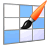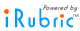# iRubric: EDU 358 Final Assessment Rubric

 find rubric
 delete .rubinfoitem { padding:5px; white-space: nowrap; } EDU 358 Final Assessment Rubric  Scoring Rubric Development - Liner Equation functions Rubric Code: L22542X Ready to use Public Rubric Subject: Math   Type: Assessment   Grade Levels: 6-8Linear Equations Distinguished 4 pts Proficient 3.6 pts Basic 3.2 pts Below Expectations 1.5 pts Non-Performance 0 pts Identify coordinate points in (x,y) format. Distinguished Correctly identifies two coordinate points as "x" and "y" in the (x,y) format. Demonstrates mastery of knowledge with analysis. Proficient Correctly identifies two coordinate points as "x" and "y" in the (x,y) format. Shows work to support evidence of knowledge. Basic Correctly identifies two coordinate points as "x" and "y" in the (x,y) format. Below Expectations Does not correctly identify two coordinate points as "x" and "y" in the (x,y) format. Non-Performance No evidence of work. Graph two sequential (x,y) points. Distinguished Accurately plots two (x,y) coordinates on a graph and properly connects them to visualize the slope of the line. Demonstrates mastery of knowledge with analysis. Proficient Accurately plots two (x,y) coordinates on a graph and properly connects them to visualize the slope of the line. Shows work to support evidence of knowledge. Basic Accurately plots two (x,y) coordinates on a graph and properly connects them to visualize the slope of the line. Below Expectations Does not accurately plot two (x,y) coordinates on a graph or connect them to visualize the slope of the line. Non-Performance No evidence of work. Identify the y-intercept and calculate the slope. Distinguished Accurately identifies the y-intercept of a line (b) and calculates the slope (m). Demonstrates mastery of knowledge with analysis. Proficient Accurately identifies the y-intercept of a line (b) and calculates the slope (m). Shows work to support evidence of knowledge. Basic Accurately identifies the y-intercept of a line (b) and calculates the slope (m). Below Expectations Does not accurately identifies the y-intercept of a line (b) and calculates the slope (m). Non-Performance No evidence of work. Interpret y=mx+b by identifying the variables. Distinguished Correctly interprets y=mx+b equation by correctly identifying the variables 100% accuracy (4 of 4). Demonstrates mastery of knowledge with analysis. Proficient Correctly interprets y=mx+b equation by correctly identifying the variables 100% accuracy (4 of 4). Shows work to support evidence of knowledge. Basic Correctly interprets y=mx+b equation by correctly identifying the variables 75% accuracy (3 of 4). Below Expectations Does not correctly interpret y=mx+b equation. Only identifies 2 or less variables correctly. Non-Performance No evidence of work. Produce a final equation in slop-intercept form. Distinguished Accurately produces a final equation in slope-intercept form with the the correctly identified y-intercept (b) and the correctly calculated slope (B) of two given coordinate points. Demonstrates mastery of knowledge with analysis. Proficient Accurately produces a final equation in slope-intercept form with the the correctly identified y-intercept (b) and the correctly calculated slope (B) of two given coordinate points. Shows work to support evidence of knowledge. Basic Accurately produces a final equation in slope-intercept form with the the correctly identified y-intercept (b) and the correctly calculated slope (B) of two given coordinate points. Below Expectations Does not accurately produce a final equation in slope-intercept form with the the correctly identified y-intercept (b) and the correctly calculated slope (B) of two given coordinate points. Non-Performance No evidence of work.

Subjects:

Types:

You may also be interested in:

Do more with this rubric:

Preview this rubric.

Modify this rubric.

Make a copy of this rubric and begin editing the copy.

Show a printable version of this rubric.

Add this rubric to multiple categories.

Bookmark this rubric for future reference.
Assess

Test this rubric or perform an ad-hoc assessment.

Build a gradebook to assess students.

Apply this rubric to any object and invite others to assess.
Share

Email this rubric to a friend.

Discuss this rubric with other members.

Do more with rubrics than ever imagined possible.

Only with iRubrictm.